### 9.3. Distance Map

#### 9.3.1. Overview

Each pixel in the image is replaced with a gray value dependent on the distance to the nearest obstacle pixel, generally a boundary pixel. Different methods can be used to calculate the distance.

#### 9.3.2. Activating the filter

This filter is found in the image window menu under FiltersGenericDistance Map….

#### 9.3.3. Options

شكل 17.181. Distance Map options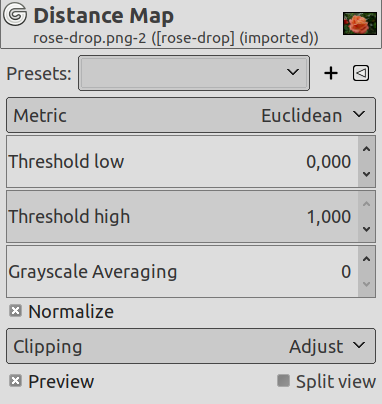Presets, Input Type, Clipping, Blending Options, Preview, Split view
ياداشتThese options are described in قسمت 2, “Common Features” .
Metric

Metric is a topology term. Three methods are available:

• Euclidean default: the distance is a straight line.

• Manhattan: the distance is the sum of the one-dimensional distances along the X and Y axes.

• Chebyshev: the distance is the maximum of the one-dimensional distances along the X and Y axes.

Edge handling

This defines how areas outside the input are considered when calculating distance. Choices are: Below threshold and Above threshold.

Threshold low

Default is 0. Increasing this value selects higher lightness pixels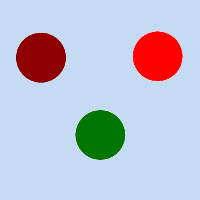Original image for examples. Threshold low will be progressively increased. Lightness: dark red = 0.070; bright red = 0.223; green = 0.133

شكل 17.182. Threshold low example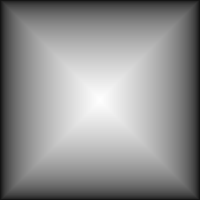Threshold low = 0: only border distances are visible.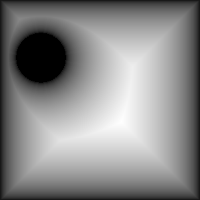Threshold low = 0.070: dark red circle appears.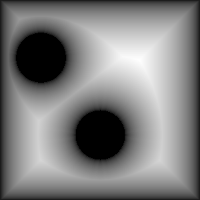Threshold low = 0.133: green circle appears.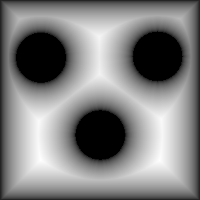Threshold low = 0.223: bright red circle appears.

Threshold high

Default is 1. Decreasing this value makes result darker.

Grayscale Averaging

Number of computations for grayscale averaging.

Normalize

This option is checked by default. If you uncheck it, no blur is created.

شكل 17.183. Normalize exampleNormalize option checked.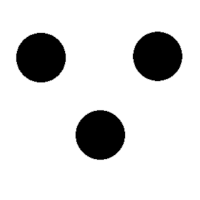Normalize option unchecked.

#### 9.3.4. Using Distance Map filter

Searching for an example using Distance map filter in image processing...